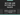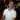# VBA Ranges - Getting and Setting Cell Values

July 23, 2017

In the previous post, we introduced the VBA Range object. This gave us the foundation of working with Ranges in VBA. In today’s post, I’d like to discuss how to get and set cell values through VBA. This will continue to build up our understanding of the VBA Range object and how to use it. There are several ways you can get and set cell values with VBA and I’ll do my best to cover all the necessities, but at the same time keeping it short and to the point. Let’s get started.

## Getting Cell Values

To get a cell’s value in VBA, we need to refer to it with the Range object and then call the `.Value` property.

We’ll use the following spreadsheet for our example. It’s a simple table with some names in it.

To get the value from cell `A2` you can use this code snippet:

``````Option Explicit

Public Sub GetCellValue()
Dim val As String
val = Range("A2").Value
Debug.Print val
End Sub``````

This will take cell `A2` and put it in the variable `val`. Then we print out the value in the Immediate Window (which the value in our example is `Joseph`).

You can also set the range to a variable and access the value from that variable as well:

``````Option Explicit

Public Sub GetCellValue()
Dim cell As Range
Set cell = Range("A2")
Debug.Print cell.Value
End Sub``````

### What happens if you use .Value on a set of cells?

Let’s change our previous code snippet to the following:

``````Option Explicit

Public Sub GetCellValue()
Dim cell As Range
Set cell = Range("A2:A5")
Debug.Print cell.Value ' will throw an error "Type Mismatch"
End Sub``````

If you run this code, you will get an error stating that there is a type mismatch.

What’s going on here?

The problem is that when you work with a set of cells, `.Value` can only return a single value. So when we ask VBA to return `.Value` on our variable (which refers to multiple cells), the `.Value` property doesn’t know which cell we are referring to.

### How do you get a single cell from a set of cells?

In order to use `.Value` to get a value from a cell, we need to refer to a single cell from the range of cells in our variable. The way we do that is with the `Cells()` VBA function.

#### The Range.Cells Function

The `Cells()` function is a way to take a range of cells and return a single cell from the set. Here is the function defined:

``Cells(row_number, column_number)``
Parameter Type Definition
`row_number` Integer The row number from within the range that you want to refer to.
`column_number` Integer The column number from within the range that you want to refer to.

Take a look at the following code:

``````Option Explicit

Public Sub GetCellValue()
Dim cellRange As Range
Set cellRange = Range("A2:A5")
Debug.Print cellRange.cells(1, 1).Value
End Sub``````

Here we took the range of `A2:A5` and referred to row 1 column 1. Since the range variable `cellRange` refers to `A2:A5`, the first row is row `2` and the first column is `A`.

BE CAREFUL!

When using the `Cells()` function, remember that row 1 and column 1 represent the top-left most cell within the range that the `Cells()` function is working on. If your range is `A1:D5`, then `Cells(1, 1)` will refer to `A1`, but if your range is `B2:D6`, then `Cells(1, 1)` refers to `B2`.

Ok, that covers getting cell values from range objects, now let’s discuss setting cell values with range objects.## Setting Cell Values

In order to set a cell’s value, you can use the same `.Value` property when referring to a cell. In this example, we’ll take `A2`’s value and change it from `Joseph` to `John`:

``````Option Explicit

Public Sub SetCellValue()
Dim cellRange As Range
Set cellRange = Range("A2")
cellRange.Value = "John"
Debug.Print cellRange.Value
End Sub``````

First we set the variable `cellRange` to `A2`. Then we said `cellRange.Value = "John"` which changes the variable’s `.Value` property. Remember, though, that the variable is a reference to cell `A2`, so whatever you do to that variable, you also do to cell `A2` in the worksheet. Finally, we output the value of `A2` into the Immediate Window to see that it changed.

We can also see the value changed in the worksheet after we run this code:

### How do you set multiple cells’ values?

Remember how I said that you can only read from one cell using `.Value`? Well, when setting values, you can actually set multiple cells at one time by using `.Value`. Take a look at the following code:

``````Option Explicit

Public Sub SetCellValue()
Dim cellRange As Range
Set cellRange = Range("A2:A5")
cellRange.Value = "John" ' Will set ALL values in the range to "John"
End Sub``````

If you ran this code, it would set all `A2:A5`’s cells to `John`:

…whoops.

Well, maybe you’d actually want to do this for some other scenarios, like when you want a bunch of cells to repeat a value.

Let’s take a real example for a second. Let’s say we have two columns, `First Name` and `Last Name`. We want to take the `Last Name` column and place its value after the `First Name`’s value; essentially combining the values to make a single `Name` column.

Here’s our sample data:

Our task is to combine the first and last name columns and place the result in column `A`. How do we do that?

One solution is to loop through cells `A2` through `A5` and then set that cell’s value to its own value, plus a space, plus the last name of the cell right next to it.

Sounds easy enough, let’s code it up:

``````Option Explicit

Public Sub SetCellValues()
Dim names As Range
Set names = Range("A2:A5")

Dim cell As Range

For Each cell In names
cell.Value = cell.Value & " " & cell.Offset(0, 1).Value
Next cell
End Sub``````

Let’s step through the code.

• First, we create a variable called `names`. Then, we set that to range `A2:A5`.
• Next, we create a variable called `cell`. This is going to be a temporary variable that will change with each iteration of the loop.
• Then, we create the loop. Here, we’re looping through the `names` range object and setting the current item to the `cell` variable. This means that each time we run through the loop, `cell` represents a single range object.

*The first time the loop is run, `cell` is set to `A2`. Then, `A3`, next `A4`, and finally `A5`. After that, there are no more cells to go through in the `names` variable, so the loop ends.

• I’ll go over how to loop through ranges in a future post since this post is already long enough!
• Now we’re ready to combine the first and last names. How we do that is with another Range function called `Offset(_rows_, _columns_)`. The idea with this function is that if you’re on a cell like `A2` and you say `cell.Offset(0, 1)` what we’re really saying is “move over one column to the right”. This puts us on cell `B2`. That’s how we’re able to get the last name in our example.

• I’ll discuss how to use the `Offset()` function in more detail in a future post. Again, this post has gone on long enough.

Here are the results of the code after we run it:

From here, we could change the `A1` cell to just `Name` and delete column `B` altogether.

## Getting and Setting Cell Values from a Named Range or Table Name

One last thing I’d like to touch on is when you use the `Range()` function, you can use a named range or table name instead of a range like `A2:A5`. In our first example, our data is in a table named `Table1`. To refer to the data of the table, we could use the following:

``````Option Explicit

Public Sub GetCellValue()
Dim cellRange As Range
Set cellRange = Range("Table1")
Debug.Print cellRange.cells(1, 1).Value
End Sub``````

And to refer to the entire table, we can leverage structured references like so:

``````Option Explicit

Public Sub GetCellValue()
Dim cellRange As Range
Set cellRange = Range("Table1[#All]")
Debug.Print cellRange.cells(1, 1).Value
End Sub``````

This will return `A1`’s value “Name” since the table starts in `A1`.

## What’s next?

Honestly, there is so much to discuss with range objects in VBA. I’ll be touching on many more topics regarding ranges in VBA in upcoming posts such as:

• Modifying cell colors
• Finding cells by their text values
• Filtering data
• Getting the last row in a range (you need this more often than you think)

I’ll come back to this post and put links to these posts as I create them.

If you enjoyed this content, please share and subscribe!

Wow, you read the whole article! You know, people who make it this far are true learners. And clearly, you value learning. Would you like to learn more about Excel? Please consider supporting me by buying me a coffee (it takes a lot of coffee to write these articles!).Written by Joseph who loves teaching about Excel.